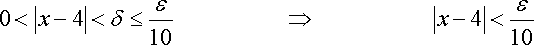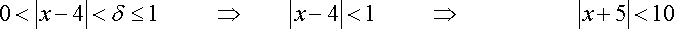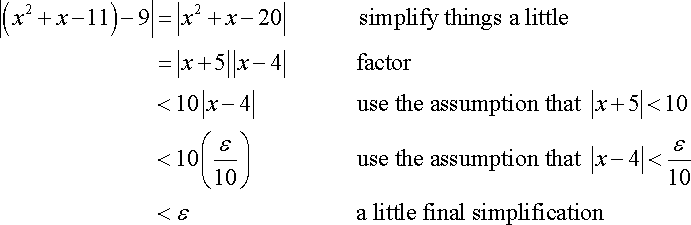# What is being Done in This proof of Limits?## Homework Statement

source:
http://tutorial.math.lamar.edu/Classes/CalcI/DefnOfLimit.aspx

If so, can you start by explaining what you think they're doing? And can you explain what you don't get??

If so, can you start by explaining what you think they're doing? And can you explain what you don't get??

This is another proof

This is another proof

It is very similar. So please, tell us what you think first.

It is very similar. So please, tell us what you think first.In this part they arrive to the conclusion that Delta have to be < ε/10.

in the next step they arrive to the same conclusion. If δ <1 then δ<10. ( I may be wrong)

The next step is what become problematic for me to understand.

Mark44
Mentor
This problem is qualitatively different from the other one. In the earlier problem, the function was linear. Here the function is a quadratic.

In the second line, which is what I believe you're asking about, they make the assumption that ##\delta < 1##. Then if ##|x - 4 | < \delta < 1##, they can say that x will be between 3 and 5. Note that I'm ignoring the part where it says 0 < |x - 4|. All this does is eliminate the possibility of x being equal to 4.

Since 3 < x < 5, the largest that |x + 5| can be is 10. From this, they can write
## |x + 5||x - 4| < 10|x - 4|##
If we take ##\delta = \epsilon/10##, then when ##|x - 4| < \delta##, it will follow that
##|x + 5||x - 4| < 10|x - 4| < 10 * \delta < 10 * \epsilon/10 = \epsilon ##